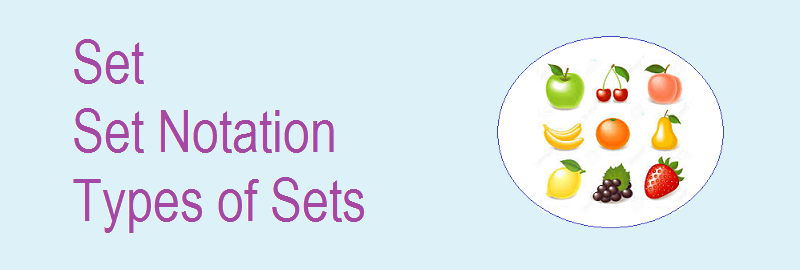# Set | Set Notation | Types of Sets## Set | Set Notation | Types of Sets

A set is the collection of well defined items or objects. The term ‘well defined objects’ is meant for those objects or members which we can identify or pick up from the given collection. If we can not identify or pick up the objects or members from the given collection, then it is not a well defined collection and hence not a set.

********************

10 Math Problems officially announces the release of Quick Math Solver, an Android App on the Google Play Store for students around the world.

********************

Here are some examples of the set given in the pictures below:
The objects of a set are called members or elements of the set.

Some examples of well defined collections:
i.    The collection of odd numbers less than 10.
ii.   The collection of first 5 letters of the English alphabet.

And, not well defined collections:
i.    The collection of tall students in your class.
ii.   The collection of difficult problems in mathematics.

### Set Notation

Sets are denoted by the capital letters of the English alphabets A, B, C, D, … etc. The objects which form a set are called the members or elements of that set. The members or elements of a set are separated by commas (,) and enclosed in curly brackets { }. Here are some examples of sets.

i.    V = {a, e, I, o, u}
ii.   F = {Factors of 18}
iii.  Z = {wild animals}

In the above example (i), the element e lies in the set V, we write e V, but m is not in V, we write m V. Thus the symbol and are used for the element belonging to and not belonging to the set.

### Types of sets

According to the numbers of elements, there are four different types of sets:
1.   Empty (Null) set: A set having no elements is called an empty or null set. For example, A = {Set of boys having 3 legs}, B = {Set of natural numbers less than 1} etc. It is denoted by or { }.
2.   Singleton (Unit) set: A set having only one element is called a singleton or unit set. For example, P = {5}, Q = {The set of highest peak of the mountains in the world} etc.
3.   Finite set: A set having a finite number of elements is called a finite set. For example, M = {2, 3, 5, 7}, N = {a, b, c, ………, x, y, z} etc.
4.   Infinite set: A set having infinite number of elements is called an infinite set. For example, set of whole numbers W = {0, 1, 2, 3, ………}, set of even numbers E = {2, 4, 6, 8, ………} etc.

### Workout Examples

Example 1: Identify which of the following sets are finite, infinite, null or singleton sets.
b.   B = {Set of odd numbers}
c.   C = { Set of factors of 12}
d.   D = {A set of multiples of 4 less than 50}
e.   E = {A set of natural numbers between 2 and 3}
f.    F = {A set of animals with one horn}
g.   G = { A set of even prime numbers}

Solution:
a.   Finite set
b.   Infinite set
c.   Finite set
d.   Finite set
e.   Empty set
f.    Singleton set
g.   Singleton set

You can comment your questions or problems regarding the set, set notation or types of sets here.